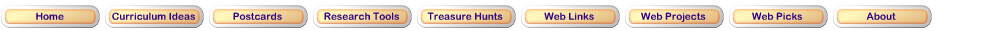Step 1: Create Pictures of Percents Less Than 1With a partner, use the Percents applet from Math Playground to create pictures of each of the pairs of percents. After you’ve done the first four examples, you will be asked questions a and b shown here, so keep them in mind as you create your pictures: Instructions for this Activity. Why do each of the pairs of pictures look the same? What is different about the two pictures? You will see percents less than 1 written as either decimals or fractions. Since the applet will only let you enter a decimal number, remember to convert the fraction percents into a decimal before entering them in the applet.Step 2: Put Percents in OrderWith a partner, use the Percents applet to try to put some percents in order from least to greatest. Instructioms for this Activity.Step 3: Understanding Sizes of PercentsWith a partner, use the Percents applet to explore the following questions about percents less than 1. Instructions for this Activity.Step 4: Extension Problem Did you know there are 20 states in the United States that have less than 1% of the US Population each? In this problem, “Estimating Populations of U.S. States,” you will practice your estimation skills as you estimate how many people live in states that have less than 1% of the US population living in them. To do this problem, you will need to know: 1) how to estimate; 2) how to find a fraction of a number; and 3) how to round off a number. This is a problem for students who love a challenge. Print out the worksheet for “Estimating Populations of U.S. States.” Pull up some benchmark numbers that will help you with this problem. As a fun trivia question, see how many of the 20 states you can name without looking at the answer key!